# 一组关于非奇异H-矩阵的细分迭代判别新条件A Set of New Criteria for the Iterative Discrimination of Subdivision of Nonsingular H-Matrices

• 全文下载: PDF(528KB)    PP.50-59   DOI: 10.12677/AAM.2020.91007
• 下载量: 28  浏览量: 58   国家自然科学基金支持

In this paper, we produced a set of new conditions for subdivided and iterative criteria of nonsingular H-matrices by the method of subdivided region and selected iterative coefficient, based on the nonsingular H-matrix and α-diagonally dominant matrix the relationship between diagonally dominant matrices. These conditions improved some recent results. Finally, several numerical examples were given to illustrate their validity.

1. 引言

${N}_{1}=\left\{i\in N:0<|{a}_{ii}|<\alpha {R}_{i}+\left(1-\alpha \right){C}_{i}\right\}$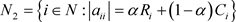${N}_{3}=\left\{i\in N:|{a}_{ii}|>\alpha {R}_{i}\left(A\right)+\left(1-\alpha \right){C}_{i}\left(A\right)\right\}$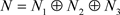${N}_{3}=\varnothing$，则 $A\notin \stackrel{˜}{D}$；若 ${N}_{1}\cup {N}_{2}=\varnothing$，显然 $A\in \stackrel{˜}{D}$。为使所论内容有意义，以下假设： ${N}_{1}\oplus {N}_{2}\ne \varnothing$${N}_{3}\ne \varnothing$

$\forall i\in {N}_{1}^{\left(k\right)},k=1,2,\cdots ,m$ 时， ${x}_{1i}^{\left(k\right)}=\frac{k|{a}_{ii}|}{m\left[\alpha {R}_{i}+\left(1-\alpha \right){C}_{i}\right]}$

$\forall i\in {N}_{2},p\ge 1$ 时， ${x}_{2i}=\frac{1}{pm}$

$\forall i\in {N}_{3}$ 时， ${r}_{0}=1$

${r}_{1}=\underset{i\in {N}_{3}}{\mathrm{max}}\left\{\frac{\alpha {R}_{i}+\left(1-\alpha \right){C}_{i}}{|{a}_{ii}|}\right\}$

${r}_{l+1}=\underset{i\in {N}_{3}}{\mathrm{max}}\left\{\frac{\alpha \left[\underset{k=1}{\overset{m}{\sum }}\left(\underset{t\in {N}_{1}^{\left(k\right)}}{\sum }|{a}_{it}|{x}_{1i}^{\left(k\right)}\right)+\alpha \underset{t\in {N}_{2}}{\sum }|{a}_{it}|{x}_{2i}+{r}_{l}\underset{t\in {N}_{3},t\ne i}{\sum }|{a}_{it}|\right]+\left(1-\alpha \right){C}_{i}}{|{a}_{ii}|}\right\},l\in {Z}^{+}$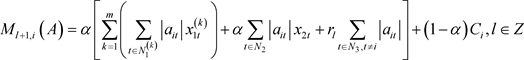${\delta }_{l+1,i}=\frac{{M}_{l+1,i}\left(A\right)}{|{a}_{ii}|},l\in Z$

${W}_{l+1,i}\left(A\right)=\frac{\alpha \left[\underset{k=1}{\overset{m}{\sum }}\left(\underset{t\in {N}_{1}^{\left(k\right)}}{\sum }|{a}_{it}|{x}_{1t}^{\left(k\right)}\right)+\underset{t\in {N}_{2}}{\sum }|{a}_{it}|{x}_{2t}\right]+\left(1-\alpha \right){C}_{i}}{{M}_{l+1,i}\left(A\right)-\alpha \underset{t\in {N}_{3},t\ne i}{\sum }|{a}_{it}|{\delta }_{l+1,i}},{h}_{l}=\underset{i\in {N}_{3}}{\mathrm{max}}{W}_{l+1,i}\left(A\right),l\in Z$

$|{a}_{ii}|{x}_{1i}^{\left(k\right)}>\alpha \left[\underset{k=1}{\overset{m}{\sum }}\left(\underset{t\in {N}_{1}^{\left(k\right)},t\ne i}{\sum }|{a}_{it}|{x}_{1t}^{\left(k\right)}\right)+\underset{t\in {N}_{2}}{\sum }|{a}_{it}|{x}_{2t}+{h}_{{l}_{0}}\underset{t\in {N}_{3}}{\sum }|{a}_{it}|{\delta }_{{l}_{0}+1,t}\right]+\left(1-\alpha \right){C}_{i}{x}_{1i}^{\left(k\right)},\text{\hspace{0.17em}}\forall i\in {N}_{1}^{\left(k\right)};k=1,2,\cdots ,m$

$|{a}_{ii}|{x}_{2i}>\alpha \left[\underset{k=1}{\overset{m}{\sum }}\left(\underset{t\in {N}_{1}^{\left(k\right)}}{\sum }|{a}_{it}|{x}_{1t}^{\left(k\right)}\right)+\underset{t\in {N}_{2},t\ne i}{\sum }|{a}_{it}|{x}_{2t}+{h}_{{l}_{0}}\underset{t\in {N}_{3}}{\sum }|{a}_{it}|{\delta }_{{l}_{0}+1,t}\right]+\left(1-\alpha \right){C}_{i}{x}_{2i},\text{\hspace{0.17em}}\text{\hspace{0.17em}}\forall i\in {N}_{2}$

2. 主要结果

$A=\left({a}_{ij}\right)\in {M}_{n}\left(C\right)$$\alpha \in \left(0,1\right]$，将不占优行的下标区间划分为m个区间，即 ${N}_{1}={N}_{1}^{\left(1\right)}\cup {N}_{1}^{\left(2\right)}\cup \cdots \cup {N}_{1}^{\left(m\right)}$，其中m是任意正整数。

${N}_{1}^{\left(1\right)}=\left\{i\in N:0<|{a}_{ii}|<\frac{1}{m}\left[\alpha {R}_{i}+\left(1-\alpha \right){C}_{i}\right]\right\}$

${N}_{1}^{\left(k\right)}=\left\{i\in N:\frac{k-1}{m}\left[\alpha {R}_{i}+\left(1-\alpha \right){C}_{i}\right]\le |{a}_{ii}|<\frac{k}{m}\left[\alpha {R}_{i}+\left(1-\alpha \right){C}_{i}\right]\right\},k=2,3,\cdots ,m$

$\forall i\in {N}_{1}^{\left(k\right)}$$k=1,2,\cdots ,m$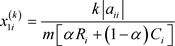$\forall i\in {N}_{2}$$p\ge 1$${x}_{2i}=\frac{1}{pm}$

$\forall i\in {N}_{3}$$k=1,2,\cdots ,m$$l=1,2,\cdots$

${\Omega }_{i}^{\left(0\right)}={r}_{0}=1$${r}_{l}=\underset{i\in {N}_{3}}{\mathrm{max}}\left(\frac{\alpha \underset{t\in {N}_{1}}{\sum }|{a}_{it}|+\alpha \underset{t\in {N}_{2}}{\sum }|{a}_{it}|+\left(1-\alpha \right){C}_{i}}{|{a}_{ii}|-\alpha \underset{t\in {N}_{3},t\ne i}{\sum }|{a}_{it}|{\Omega }_{t}^{\left(l-1\right)}}\right)$

${P}_{l,i}=\alpha \left(\underset{t\in {N}_{1}}{\sum }|{a}_{it}|+\underset{t\in {N}_{2}}{\sum }|{a}_{it}|+{r}_{l}\underset{t\in {N}_{3},t\ne i}{\sum }|{a}_{it}|{\Omega }_{t}^{\left(l-1\right)}\right)+\left(1-\alpha \right){C}_{i}$

${\Omega }_{i}^{\left(l\right)}=\frac{{P}_{l,i}}{|{a}_{ii}|}$${H}_{l}=\underset{i\in {N}_{3}}{\mathrm{max}}{M}_{l,i}$

2.1. 定理1

$A\in {M}_{n}\left(C\right)$$\alpha \in \left(0,1\right]$，若存在 ${l}_{0}\in Z,p\ge 1$，使满足：

$\begin{array}{l}|{a}_{ii}|{x}_{1i}^{\left(k\right)}>\alpha \left[\underset{k=1}{\overset{m}{\sum }}\left(\underset{t\in {N}_{1}^{\left(k\right)},t\ne i}{\sum }|{a}_{it}|{x}_{1t}^{\left(k\right)}\right)+\underset{t\in {N}_{2}}{\sum }|{a}_{it}|{x}_{2t}+{H}_{{l}_{0}}\underset{t\in {N}_{3}}{\sum }|{a}_{it}|{\Omega }_{t}^{\left({l}_{0}\right)}\right]\\ \text{\hspace{0.17em}}\text{\hspace{0.17em}}\text{\hspace{0.17em}}\text{\hspace{0.17em}}\text{\hspace{0.17em}}\text{\hspace{0.17em}}\text{\hspace{0.17em}}\text{\hspace{0.17em}}\text{\hspace{0.17em}}\text{\hspace{0.17em}}\text{\hspace{0.17em}}\text{\hspace{0.17em}}\text{\hspace{0.17em}}+\left(1-\alpha \right){C}_{i}{x}_{1i}^{\left(k\right)},\text{\hspace{0.17em}}\text{\hspace{0.17em}}\forall i\in {N}_{1}^{\left(k\right)};k=1,2,\cdots ,m\end{array}$ (1)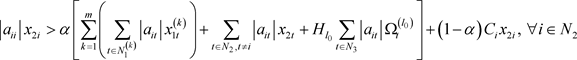(2)

$\begin{array}{c}{M}_{l,i}=\frac{\alpha \underset{k=1}{\overset{m}{\sum }}\left(\underset{t\in {N}_{1}^{\left(k\right)}}{\sum }|{a}_{it}|{x}_{1t}^{\left(k\right)}\right)+\alpha \underset{t\in {N}_{2}}{\sum }|{a}_{it}|{x}_{2t}+\left(1-\alpha \right){C}_{i}}{{P}_{l,i}-\alpha {r}_{l}\underset{t\in {N}_{3},t\ne i}{\sum }|{a}_{it}|{\Omega }_{t}^{\left(l\right)}}\\ \le \frac{\alpha \underset{k=1}{\overset{m}{\sum }}\left(\underset{t\in {N}_{1}^{\left(k\right)}}{\sum }|{a}_{it}|{x}_{1t}^{\left(k\right)}\right)+\alpha \underset{t\in {N}_{2}}{\sum }|{a}_{it}|{x}_{2t}+\left(1-\alpha \right){C}_{i}}{{P}_{l,i}-\alpha {r}_{l}\underset{t\in {N}_{3},t\ne i}{\sum }|{a}_{it}|{\Omega }_{t}^{\left(l-1\right)}}\\ \le \frac{\alpha \underset{t\in {N}_{1}}{\sum }|{a}_{it}|+\alpha \underset{t\in {N}_{2}}{\sum }|{a}_{it}|+\left(1-\alpha \right){C}_{i}}{{P}_{l,i}-\alpha {r}_{l}\underset{t\in {N}_{3},t\ne i}{\sum }|{a}_{it}|{\Omega }_{t}^{\left(l-1\right)}}=1\end{array}$

$\begin{array}{l}\epsilon \alpha \underset{t\in {N}_{3}}{\sum }|{a}_{it}|<|{a}_{ii}|{x}_{1i}^{\left(k\right)}-\alpha \left[\underset{k=1}{\overset{m}{\sum }}\left(\underset{t\in {N}_{1}^{\left(k\right)}}{\sum }|{a}_{it}|{x}_{1t}^{\left(k\right)}\right)+\underset{t\in {N}_{2}}{\sum }|{a}_{it}|{x}_{2t}+{H}_{{l}_{0}}\underset{t\in {N}_{3},t\ne i}{\sum }|{a}_{it}|{\Omega }_{t}^{\left({l}_{0}\right)}\right]\\ \text{\hspace{0.17em}}\text{\hspace{0.17em}}\text{\hspace{0.17em}}\text{\hspace{0.17em}}\text{\hspace{0.17em}}\text{\hspace{0.17em}}\text{\hspace{0.17em}}\text{\hspace{0.17em}}\text{\hspace{0.17em}}\text{\hspace{0.17em}}\text{\hspace{0.17em}}\text{\hspace{0.17em}}\text{\hspace{0.17em}}\text{\hspace{0.17em}}\text{\hspace{0.17em}}\text{\hspace{0.17em}}\text{\hspace{0.17em}}-\left(1-\alpha \right){C}_{i}{x}_{1i}^{\left(k\right)},\text{\hspace{0.17em}}\forall i\in {N}_{1}^{\left(k\right)};k=1,2,\cdots ,m\end{array}$ (3)

$\epsilon \alpha \underset{t\in {N}_{3}}{\sum }|{a}_{it}|<|{a}_{ii}|{x}_{2t}-\alpha \left[\underset{k=1}{\overset{m}{\sum }}\left(\underset{t\in {N}_{1}^{k},t\ne i}{\sum }|{a}_{it}|{x}_{1t}^{\left(k\right)}\right)+\underset{t\in {N}_{2}}{\sum }|{a}_{it}|{x}_{2t}+{H}_{{l}_{0}}\underset{t\in {N}_{3}}{\sum }|{a}_{it}|{\Omega }_{t}^{\left({l}_{0}\right)}\right]-\left(1-\alpha \right){C}_{i}{x}_{2i},\text{\hspace{0.17em}}\text{\hspace{0.17em}}\forall i\in {N}_{2}$ (4)

${H}_{{l}_{0}}{P}_{{l}_{0},i}\ge \alpha \left[\underset{k=1}{\overset{m}{\sum }}\left(\underset{t\in {N}_{1}^{k}}{\sum }|{a}_{it}|{x}_{1t}^{\left(k\right)}\right)+\underset{t\in {N}_{2}}{\sum }|{a}_{it}|{x}_{2t}+{H}_{{l}_{0}}{r}_{{l}_{0}}\underset{t\in {N}_{3},t\ne i}{\sum }|{a}_{it}|{\Omega }_{t}^{\left({l}_{0}\right)}\right]+\left(1-\alpha \right){C}_{i}$

$\alpha \left[\underset{k=1}{\overset{m}{\sum }}\left(\underset{t\in {N}_{1}^{\left(k\right)}}{\sum }|{a}_{it}|{x}_{1t}^{\left(k\right)}\right)+\underset{t\in {N}_{2}}{\sum }|{a}_{it}|{x}_{2t}+{H}_{{l}_{0}}{r}_{{l}_{0}}\underset{t\in {N}_{3},t\ne i}{\sum }|{a}_{it}|{\Omega }_{t}^{\left({l}_{0}\right)}\right]+\left(1-\alpha \right){C}_{i}-{H}_{{l}_{0}}{\Omega }_{t}^{\left({l}_{0}\right)}|{a}_{ii}|\le 0$

${H}_{{l}_{0}}{\Omega }_{t}^{\left({l}_{0}\right)}|{a}_{ii}|-\alpha \left[\underset{k=1}{\overset{m}{\sum }}\left(\underset{t\in {N}_{1}^{\left(k\right)}}{\sum }|{a}_{it}|{x}_{1t}^{\left(k\right)}\right)+\underset{t\in {N}_{2}}{\sum }|{a}_{it}|{x}_{2t}+{H}_{{l}_{0}}{r}_{{l}_{0}}\underset{t\in {N}_{3},t\ne i}{\sum }|{a}_{it}|{\Omega }_{t}^{\left({l}_{0}\right)}\right]+\left(1-\alpha \right){C}_{i}\ge 0$

${r}_{{l}_{0}}={r}_{0}=1$ 时，易知上述等式依旧成立。

$\begin{array}{l}{H}_{{l}_{0}}{\Omega }_{t}^{\left({l}_{0}\right)}|{a}_{ii}|-\alpha \left[\underset{k=1}{\overset{m}{\sum }}\left(\underset{t\in {N}_{1}^{\left(k\right)}}{\sum }|{a}_{it}|{x}_{1t}^{\left(k\right)}\right)+\underset{t\in {N}_{2}}{\sum }|{a}_{it}|{x}_{2t}+{H}_{{l}_{0}}{r}_{{l}_{0}}\underset{t\in {N}_{3},t\ne i}{\sum }|{a}_{it}|{\Omega }_{t}^{\left({l}_{0}\right)}\right]+\left(1-\alpha \right){C}_{i}\\ \text{ }+\epsilon \left[|{a}_{ii}|-\alpha \underset{t\in {N}_{3},t\ne i}{\sum }|{a}_{it}|+\left(1-\alpha \right){C}_{i}\right]>0\end{array}$ (5)1) $\forall i\in {N}_{1}^{\left(k\right)}$，由(3)式得，

$\begin{array}{l}\alpha {R}_{i}\left(B\right)+\left(1-\alpha \right){C}_{i}\left(B\right)\\ =\alpha \left[\underset{k=1}{\overset{m}{\sum }}\left(\underset{t\in {N}_{1}^{\left(k\right)},t\ne i}{\sum }|{a}_{it}|{x}_{1t}^{\left(k\right)}\right)+\underset{t\in {N}_{2}}{\sum }|{a}_{it}|{x}_{2t}+\underset{t\in {N}_{3}}{\sum }|{a}_{it}|\left({H}_{{l}_{0}}{\Omega }_{t}^{\left({l}_{0}\right)}+\epsilon \right)\right]+\left(1-\alpha \right){C}_{i}\left(A\right){x}_{1i}^{\left(k\right)}\\ =\alpha \left[\underset{k=1}{\overset{m}{\sum }}\left(\underset{t\in {N}_{1}^{\left(k\right)},t\ne i}{\sum }|{a}_{it}|{x}_{1t}^{\left(k\right)}\right)+\underset{t\in {N}_{2}}{\sum }|{a}_{it}|{x}_{2t}+{H}_{{l}_{0}}\underset{t\in {N}_{3}}{\sum }|{a}_{it}|{\Omega }_{t}^{\left({l}_{0}\right)}\right]+\left(1-\alpha \right){C}_{i}\left(A\right){x}_{1i}^{\left(k\right)}+\epsilon \alpha \underset{t\in {N}_{3}}{\sum }|{a}_{it}|\\ <|{a}_{ii}|{x}_{1i}^{\left(k\right)}=|{b}_{ii}|\end{array}$

2) $\forall i\in {N}_{2}$，由(4)式得，

$\begin{array}{l}\alpha {R}_{i}\left(B\right)+\left(1-\alpha \right){C}_{i}\left(B\right)\\ =\alpha \left[\underset{k=1}{\overset{m}{\sum }}\left(\underset{t\in {N}_{1}^{\left(k\right)}}{\sum }|{a}_{it}|{x}_{1t}^{\left(k\right)}\right)+\underset{t\in {N}_{2},t\ne i}{\sum }|{a}_{it}|{x}_{2t}+\underset{t\in {N}_{3}}{\sum }|{a}_{it}|\left({H}_{{l}_{0}}{\Omega }_{t}^{\left({l}_{0}\right)}+\epsilon \right)\right]+\left(1-\alpha \right){C}_{i}\left(A\right){x}_{2i}\\ =\alpha \left[\underset{k=1}{\overset{m}{\sum }}\left(\underset{t\in {N}_{1}^{\left(k\right)}}{\sum }|{a}_{it}|{x}_{1t}^{\left(k\right)}\right)+\underset{t\in {N}_{2},t\ne i}{\sum }|{a}_{it}|{x}_{2t}+{H}_{{l}_{0}}\underset{t\in {N}_{3}}{\sum }|{a}_{it}|{\Omega }_{t}^{\left({l}_{0}\right)}\right]+\left(1-\alpha \right){C}_{i}\left(A\right){x}_{2i}+\epsilon \alpha \underset{t\in {N}_{3}}{\sum }|{a}_{it}|\\ <|{a}_{ii}|{x}_{2i}=|{b}_{ii}|\end{array}$

3) $\forall i\in {N}_{3}$，因为 ${H}_{l}\le 1$${\Omega }_{i}^{\left(l\right)}\le 1$，所以 ${H}_{{l}_{0}}{\Omega }_{t}^{\left({l}_{0}\right)}\le 1$，再由(5)式得，

$\begin{array}{l}|{b}_{ii}|-\alpha {R}_{i}\left(B\right)-\left(1-\alpha \right){C}_{i}\left(B\right)\\ =\left({H}_{{l}_{0}}{\Omega }_{t}^{\left({l}_{0}\right)}+\epsilon \right)|{a}_{ii}|-\alpha \left[\underset{k=1}{\overset{m}{\sum }}\left(\underset{t\in {N}_{1}^{\left(k\right)}}{\sum }|{a}_{it}|{x}_{1t}^{\left(k\right)}\right)+\underset{t\in {N}_{2}}{\sum }|{a}_{it}|{x}_{2t}+\underset{t\in {N}_{3},t\ne i}{\sum }|{a}_{it}|\left({H}_{{l}_{0}}{\Omega }_{t}^{\left({l}_{0}\right)}+\epsilon \right)\right]\\ \text{\hspace{0.17em}}\text{\hspace{0.17em}}\text{ }\text{ }-\left(1-\alpha \right){C}_{i}\left(A\right)\left({H}_{{l}_{0}}{\Omega }_{t}^{\left({l}_{0}\right)}+\epsilon \right)\\ =\epsilon \left[|{a}_{ii}|-\alpha \underset{t\in {N}_{3},t\ne i}{\sum }|{a}_{it}|-\left(1-\alpha \right){C}_{i}\left(A\right)\right]+{H}_{{l}_{0}}{\Omega }_{t}^{\left({l}_{0}\right)}|{a}_{ii}|\\ \text{\hspace{0.17em}}\text{\hspace{0.17em}}\text{ }\text{ }-\alpha \left[\underset{k=1}{\overset{m}{\sum }}\left(\underset{t\in {N}_{1}^{\left(k\right)}}{\sum }|{a}_{it}|{x}_{1t}^{\left(k\right)}\right)+\underset{t\in {N}_{2}}{\sum }|{a}_{it}|{x}_{2t}+{H}_{{l}_{0}}\underset{t\in {N}_{3},t\ne i}{\sum }|{a}_{it}|{\Omega }_{t}^{\left({l}_{0}\right)}\right]-\left(1-\alpha \right){C}_{i}\left(A\right){H}_{{l}_{0}}{\Omega }_{t}^{\left(l0\right)}\end{array}$

$\begin{array}{l}>\epsilon \left[|{a}_{ii}|-\alpha \underset{t\in {N}_{3},t\ne i}{\sum }|{a}_{it}|-\left(1-\alpha \right){C}_{i}\left(A\right)\right]+{H}_{{l}_{0}}{\Omega }_{t}^{\left({l}_{0}\right)}|{a}_{ii}|\\ \text{\hspace{0.17em}}\text{\hspace{0.17em}}\text{ }\text{ }-\alpha \left[\underset{k=1}{\overset{m}{\sum }}\left(\underset{t\in {N}_{1}^{\left(k\right)}}{\sum }|{a}_{it}|{x}_{1t}^{\left(k\right)}\right)+\underset{t\in {N}_{2}}{\sum }|{a}_{it}|{x}_{2t}+{H}_{{l}_{0}}\underset{t\in {N}_{3},t\ne i}{\sum }|{a}_{it}|{\Omega }_{t}^{\left({l}_{0}\right)}\right]-\left(1-\alpha \right){C}_{i}\left(A\right)\\ >0\end{array}$

2.1.1. 推论1

$A\in {M}_{n}\left(C\right)$，若存在 ${l}_{0}\in Z$，使满足

$|{a}_{ii}|{x}_{1i}>\underset{k=1}{\overset{m}{\sum }}\left(\underset{t\in {N}_{1}^{\left(k\right)},t\ne i}{\sum }|{a}_{it}|{x}_{1t}^{\left(k\right)}\right)+\underset{t\in {N}_{2}}{\sum }|{a}_{2t}|{x}_{2t}+{H}_{{l}_{0}}\underset{t\in {N}_{3}}{\sum }|{a}_{it}|{\Omega }_{t}^{\left({l}_{0}\right)},\text{\hspace{0.17em}}\text{\hspace{0.17em}}\forall i\in {N}_{1};k=1,2,\cdots ,m$

$|{a}_{ii}|{x}_{2i}^{\left(k\right)}>\underset{k=1}{\overset{m}{\sum }}\left(\underset{t\in {N}_{1}^{\left(k\right)}}{\sum }|{a}_{it}|{x}_{1t}^{\left(k\right)}\right)+\underset{t\in {N}_{2},t\ne i}{\sum }|{a}_{2t}|{x}_{2t}+{H}_{{l}_{0}}\underset{t\in {N}_{3}}{\sum }|{a}_{it}|{\Omega }_{t}^{\left({l}_{0}\right)},\text{\hspace{0.17em}}\text{\hspace{0.17em}}\forall i\in {N}_{2}^{\left(k\right)}$

2.1.2. 推论2

$A\in {M}_{n}\left(C\right)$，若存在 ${l}_{0}\in Z$，使满足

$|{a}_{ii}|\frac{|{a}_{ii}|}{{R}_{i}}>\underset{t\in {N}_{1}\cup {N}_{2},t\ne i}{\sum }|{a}_{it}|\frac{|{a}_{ii}|}{{R}_{i}}+{H}_{{l}_{0}}\underset{t\in {N}_{3}}{\sum }|{a}_{it}|{\Omega }_{t}^{\left({l}_{0}\right)},\text{\hspace{0.17em}}\text{\hspace{0.17em}}\forall i\in {N}_{1};k=1,2,\cdots ,m$

2.2. 定理2

$A\in {M}_{n}\left(C\right)$$\alpha \in \left(0,1\right]$，矩阵A不可约，若存在 ${l}_{0}\in Z,p\ge 1$，使满足

$\begin{array}{l}|{a}_{ii}|{x}_{1i}^{\left(k\right)}\ge \alpha \left[\underset{k=1}{\overset{m}{\sum }}\left(\underset{t\in {N}_{1}^{\left(k\right)},t\ne i}{\sum }|{a}_{it}|{x}_{1t}^{\left(k\right)}\right)+\underset{t\in {N}_{2}}{\sum }|{a}_{it}|{x}_{2t}+{H}_{{l}_{0}}\underset{t\in {N}_{3}}{\sum }|{a}_{it}|{\Omega }_{t}^{\left({l}_{0}\right)}\right]\\ \text{\hspace{0.17em}}\text{\hspace{0.17em}}\text{\hspace{0.17em}}\text{\hspace{0.17em}}\text{\hspace{0.17em}}\text{\hspace{0.17em}}\text{\hspace{0.17em}}\text{\hspace{0.17em}}\text{\hspace{0.17em}}\text{\hspace{0.17em}}\text{\hspace{0.17em}}\text{\hspace{0.17em}}\text{\hspace{0.17em}}+\left(1-\alpha \right){C}_{i}{x}_{1i}^{\left(k\right)},\text{\hspace{0.17em}}\text{\hspace{0.17em}}\forall i\in {N}_{1}^{\left(k\right)};k=1,2,\cdots ,m\end{array}$ (6)

$|{a}_{ii}|{x}_{2i}\ge \alpha \left[\underset{k=1}{\overset{m}{\sum }}\left(\underset{t\in {N}_{1}^{\left(k\right)}}{\sum }|{a}_{it}|{x}_{1t}^{\left(k\right)}\right)+\underset{t\in {N}_{2},t\ne i}{\sum }|{a}_{it}|{x}_{2t}+{H}_{{l}_{0}}\underset{t\in {N}_{3}}{\sum }|{a}_{it}|{\Omega }_{t}^{\left({l}_{0}\right)}\right]+\left(1-\alpha \right){C}_{i}{x}_{2i},\text{\hspace{0.17em}}\text{\hspace{0.17em}}\forall i\in {N}_{2}$ (7)

2.3. 定理3

${K}_{1}=\left\{i\in {N}_{1}^{\left(k\right)}:|{a}_{ii}|{x}_{1i}^{\left(k\right)}>\alpha \left[\underset{k=1}{\overset{m}{\sum }}\left(\underset{t\in {N}_{1}^{\left(k\right)},t\ne i}{\sum }|{a}_{it}|{x}_{1t}^{\left(k\right)}\right)+\underset{t\in {N}_{2}}{\sum }|{a}_{it}|{x}_{2t}+{H}_{{l}_{0}}\underset{t\in {N}_{3}}{\sum }|{a}_{it}|{\Omega }_{t}^{\left({l}_{0}\right)}\right]+\left(1-\alpha \right){C}_{i}{x}_{1i}^{\left(k\right)}\right\};k=1,2,\cdots ,m$

${K}_{1}=\underset{k=1}{\overset{m}{\cup }}{K}_{1}^{\left(k\right)}$

${K}_{2}=\left\{i\in {N}_{2}:|{a}_{ii}|{x}_{2i}>\alpha \left[\underset{k=1}{\overset{m}{\sum }}\left(\underset{t\in {N}_{1}^{\left(k\right)}}{\sum }|{a}_{it}|{x}_{1t}^{\left(k\right)}\right)+\underset{t\in {N}_{2},t\ne i}{\sum }|{a}_{it}|{x}_{2t}+{H}_{{l}_{0}}\underset{t\in {N}_{3}}{\sum }|{a}_{it}|{\Omega }_{t}^{\left({l}_{0}\right)}\right]+\left(1-\alpha \right){C}_{i}{x}_{2i}\right\}$

${K}_{3}=\left\{i\in {N}_{3}:|{a}_{ii}|{H}_{{l}_{0}}{\Omega }_{i}^{\left({l}_{0}\right)}>\alpha \left[\underset{k=1}{\overset{m}{\sum }}\left(\underset{t\in {N}_{1}^{\left(k\right)}}{\sum }|{a}_{it}|{x}_{1t}^{\left(k\right)}\right)+\underset{t\in {N}_{2}}{\sum }|{a}_{it}|{x}_{2t}+{H}_{{l}_{0}}\underset{t\in {N}_{3},t\ne i}{\sum }|{a}_{it}|{\Omega }_{t}^{\left({l}_{0}\right)}\right]+\left(1-\alpha \right){C}_{i}{H}_{{l}_{0}}{\Omega }_{i}^{\left({l}_{0}\right)}\right\}$

$A\in {M}_{n}\left(C\right)$$\alpha \in \left(0,1\right]$，若存在 ${l}_{0}\in Z,p\ge 1$，使满足

$\begin{array}{l}|{a}_{ii}|{x}_{1i}^{\left(k\right)}\ge \alpha \left[\underset{k=1}{\overset{m}{\sum }}\left(\underset{t\in {N}_{1}^{\left(k\right)},t\ne i}{\sum }|{a}_{it}|{x}_{1t}^{\left(k\right)}\right)+\underset{t\in {N}_{2}}{\sum }|{a}_{it}|{x}_{2t}+{H}_{{l}_{0}}\underset{t\in {N}_{3}}{\sum }|{a}_{it}|{\Omega }_{t}^{\left({l}_{0}\right)}\right]\\ \text{\hspace{0.17em}}\text{\hspace{0.17em}}\text{\hspace{0.17em}}\text{\hspace{0.17em}}\text{\hspace{0.17em}}\text{\hspace{0.17em}}\text{\hspace{0.17em}}\text{\hspace{0.17em}}\text{\hspace{0.17em}}\text{\hspace{0.17em}}\text{\hspace{0.17em}}\text{\hspace{0.17em}}\text{\hspace{0.17em}}+\left(1-\alpha \right){C}_{i}{x}_{1i}^{\left(k\right)},\text{\hspace{0.17em}}\text{\hspace{0.17em}}\forall i\in {N}_{1}^{\left(k\right)};k=1,2,\cdots ,m\end{array}$ (8)

$|{a}_{ii}|{x}_{2i}\ge \alpha \left[\underset{k=1}{\overset{m}{\sum }}\left(\underset{t\in {N}_{1}^{\left(k\right)}}{\sum }|{a}_{it}|{x}_{1t}^{\left(k\right)}\right)+\underset{t\in {N}_{2},t\ne i}{\sum }|{a}_{it}|{x}_{2t}+{H}_{{l}_{0}}\underset{t\in {N}_{3}}{\sum }|{a}_{it}|{\Omega }_{t}^{\left({l}_{0}\right)}\right]+\left(1-\alpha \right){C}_{i}{x}_{2i},\text{\hspace{0.17em}}\text{\hspace{0.17em}}\forall i\in {N}_{2}$ (9)

3. 数值实例

$A=\left(\begin{array}{ccccc}2& 0& 0& 1& 1\\ 0\text{}& 3.2& 1& 2& 3\\ 1& 1& 18& 4& 5\\ 1& 1& 3& 25& 4\\ 0& 1.5& 2& 3& 20\end{array}\right)$

${\delta }_{2,3}=0.3258,{\delta }_{2,4}=0.2951,{\delta }_{2,5}=0.4028,{h}_{1}=0.9613$ (文献  结果)；

${\Omega }_{3}^{\left(l\right)}=0.3258,{\Omega }_{4}^{\left(l\right)}=0.298,{\Omega }_{5}^{\left(l\right)}=0.4143,{H}_{1}=0.8975$ (本文结果)，时，根据

$\begin{array}{l}2.1558=3.2×0.6737=|{a}_{22}|{x}_{12}>\alpha \left[|{a}_{21}|{x}_{21}+{H}_{1}\left(|{a}_{23}|{\Omega }_{3}^{\left(l\right)}+|{a}_{24}|{\Omega }_{4}^{\left(l\right)}+|{a}_{25}|{\Omega }_{5}^{\left(l\right)}\right)\right]+\left(1-\alpha \right){C}_{2}{x}_{21}\\ =\frac{1}{2}×\left[0+0.8795×\left(0.3258+2×0.298+3×0.4143\right)\right]+\frac{1}{2}×3.5×0.6737=2.1309\end{array}$

$X=\left(\begin{array}{ccccc}0.6737& 0& 0& 0& 0\\ 0\text{}& 0.\stackrel{˙}{6}& 0& 0& 0\\ 0& 0& 0.2865& 0& 0\\ 0& 0& 0& 0.2621& 0\\ 0& 0& 0& 0& 0.3643\end{array}\right)$

NOTES

*通讯作者。

  范迎松, 陆全, 徐仲, 高慧敏. 非奇异H-矩阵的一组判定条件[J]. 高校应用数学学报A辑, 2011, 26(4): 474-480.  尹军茹, 徐仲, 陆全. 非奇H-矩阵的细分迭代判别准则[J]. 工程数学学报, 2013, 30(3): 433-441.  山瑞平, 陆全, 徐仲, 张骁. 非奇H-矩阵的一组细分迭代判定条件[J]. 应用数学学报, 2014, 37(6): 1130-1139.  庹清, 陈茜. 关于“一类非奇异H-矩阵判定的新条件”一文的注记[J]. 计算数学, 2019, 41(2): 219-224.  刘长太. 非奇异H矩阵迭代式充分条件[J]. 计算数学, 2017, 39(3): 328-336.  Gan, T.-B. and Huang, T.-Z. (2003) Simple Criteria for Nonsingular H-Matrices. Linear Algebra and Its Applications, 374, 317-326. https://doi.org/10.1016/S0024-3795(03)00646-3  Berman, A. and Plemmons, R.J. (1979) Nonnegative Matrix in the Mathematical Sciences. Academic Press, New York. https://doi.org/10.1016/B978-0-12-092250-5.50009-6  谢清明. 判定广义对角占优矩阵的几个充分条件[J]. 工程数学学报, 2006(4): 757-760.  孙玉祥. 广义对角占优矩阵的充分条件[J]. 高等学校计算数学学报, 1997(3): 216-223.